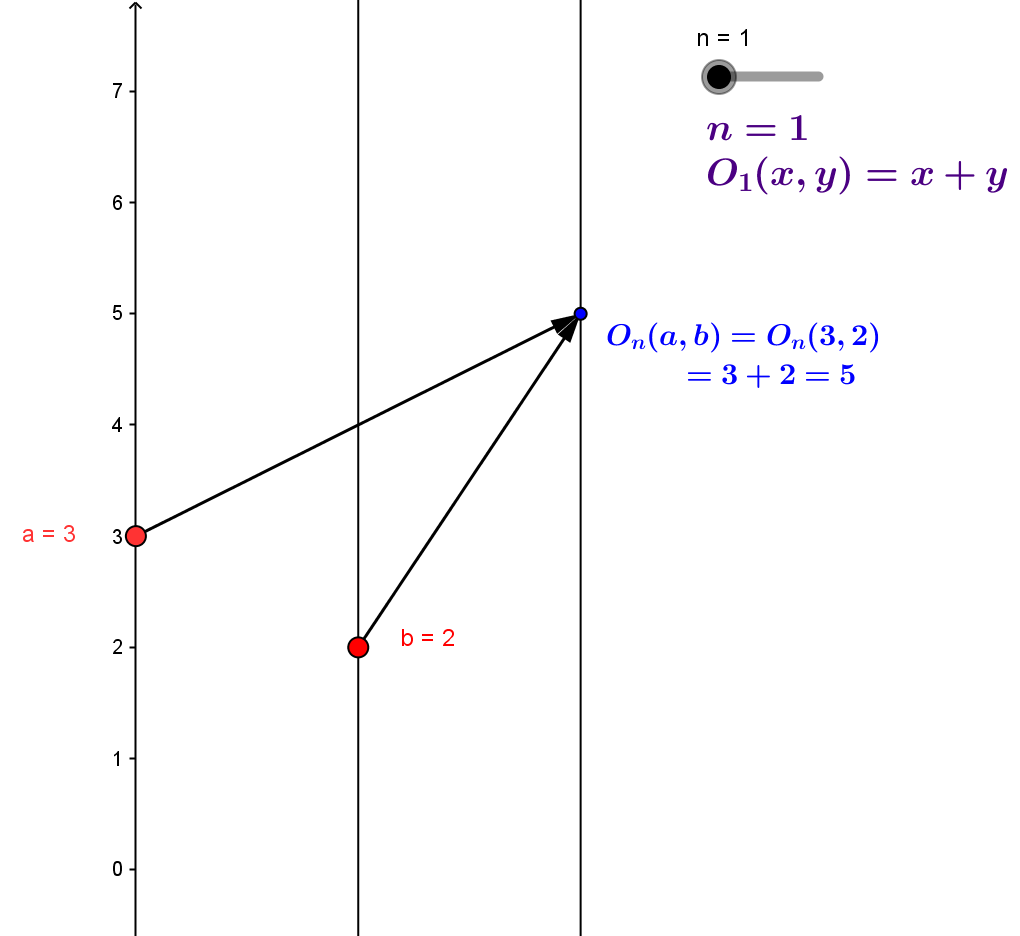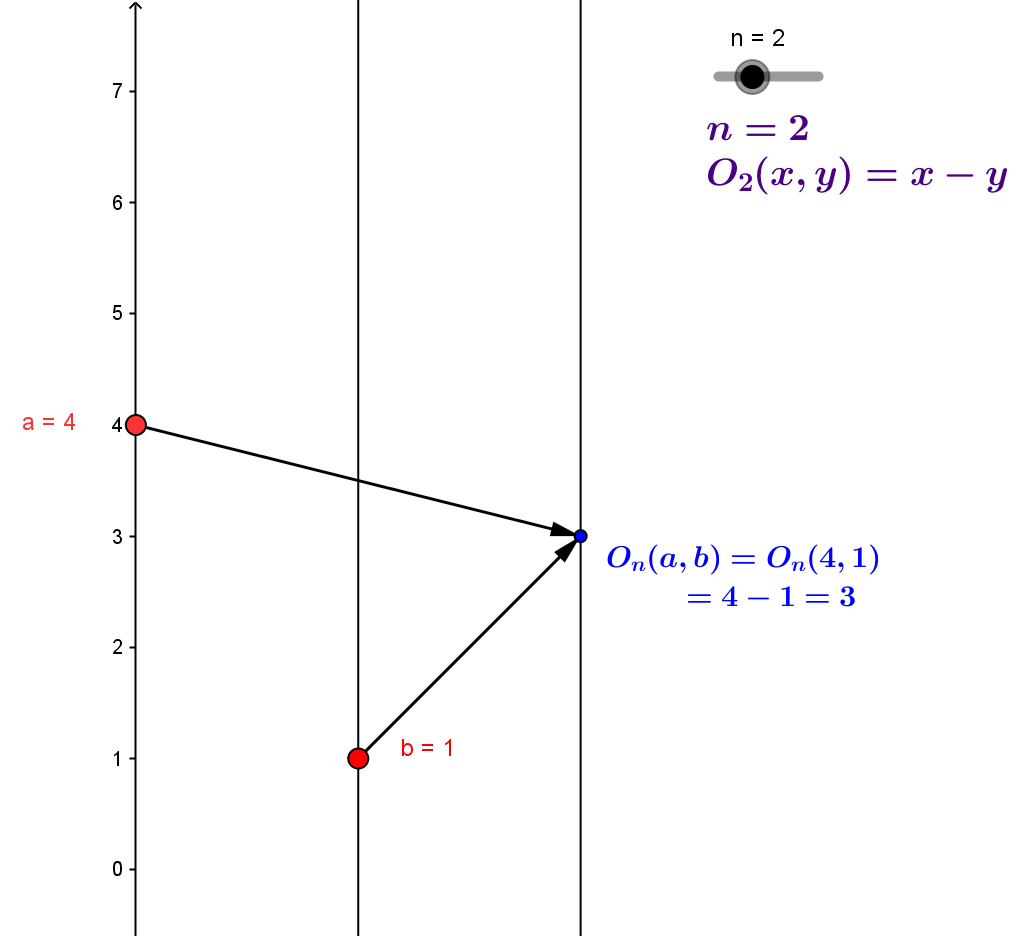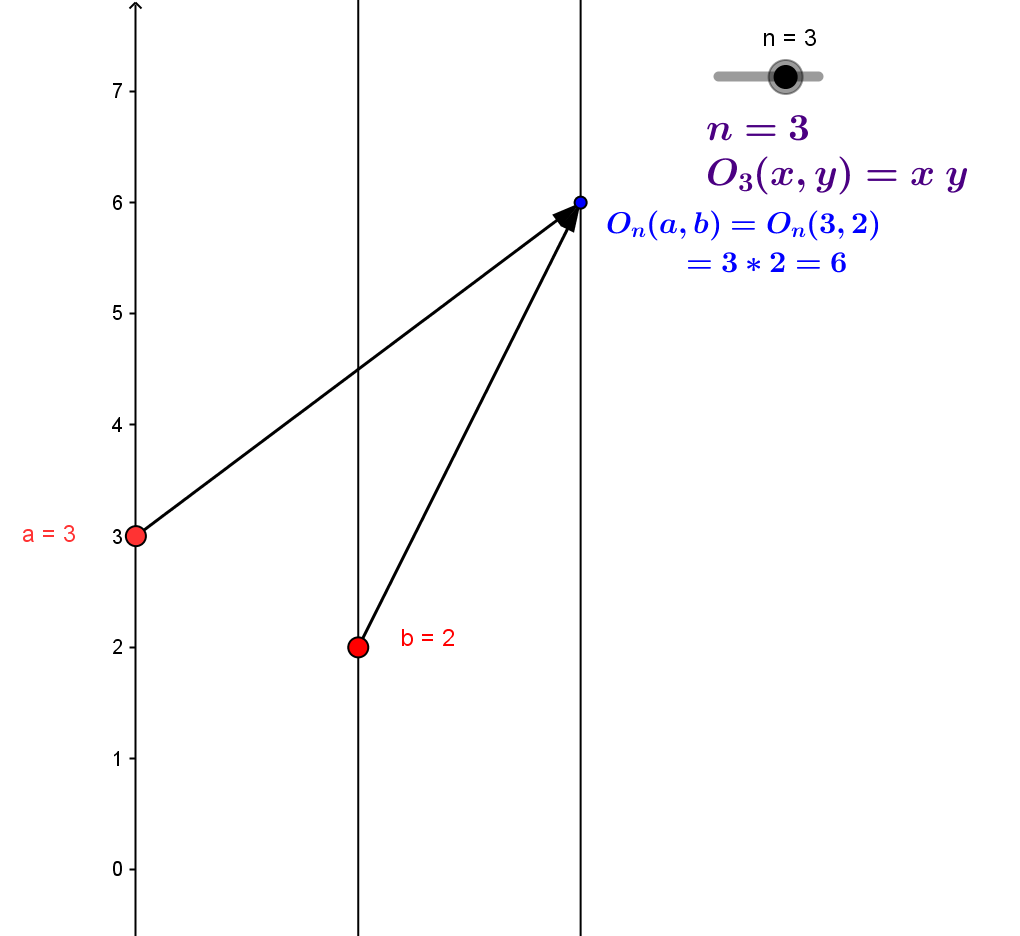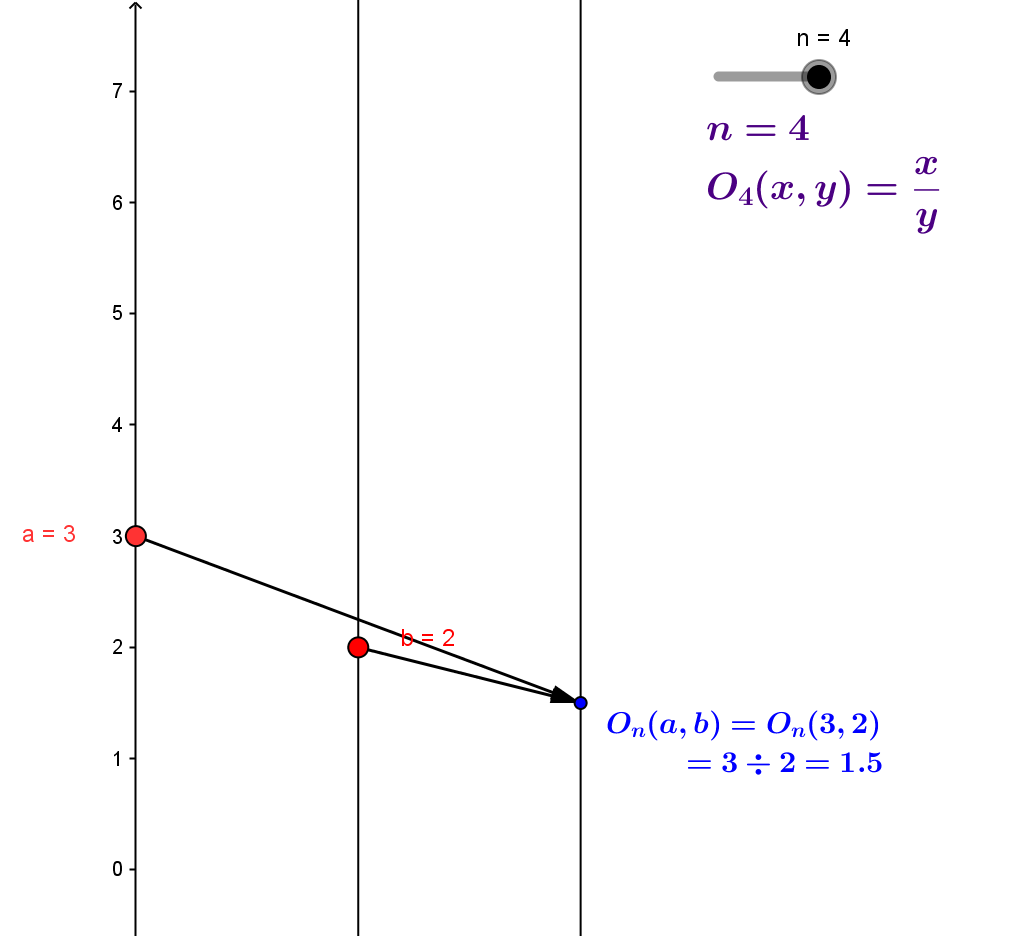AEF.AOEF Arithmetic Operations and Elementary Functions

The elementary functions are defined initially from a list of core functions.

The definition continues with a recursive formulation, building more elementary functions from previously defined elementary functions by using arithmetic operations, composition of functions, and inverting functions.
In this section we focus on visualizing the use of arithmetic operations with mapping diagrams to make sense of elementary functions formed by using arithmetic.

Visualizing Arithmetic Operations with Mapping Diagrams
There are two core binary arithmetic operations, addition $+$ and multiplication $\times$
and two secondary binary arithmetic operations, subtraction $-$ and division $\div$.
The algebraic properties of these operations are studied from early grade school, and tables are frequently used to present the common arithmetic facts. No visualization is attached to operations that connects them to graphs except much later in school when graphs of functions of two variables are introduced.

Mapping diagrams provide a simple and consistent tool for making sense of binary operations without requiring a more subtle understanding required for graphs.

One can consider a binary operation as a function of two variables, with the value of the function being the result of applying the operation to an ordered pair of numbers.
To visualize the operation (function) we use two (ordered) vertical axes for the ordered pair of numbers to which the operation (function) is applied, and a third vertical axis where the result is marked.
From a single number  on the first and second axes an arrow emanates meeting together at the number on the third axis corresponding to the result of the operation.GeoGebra Mapping Diagram for Arithmetic Operations
$n=1: +, n=2: -,n=3: \times, n=4 : \div$
Change $n$ with the slider.

The Arithmetic of Functions:Arithmetic Operations Applied to Functions

We apply an arithmetic operation, $O$, to functions, $f_1$ and $f_2$, to create a new function, $f$. Let $f(x) = O(f_1(x),f_2(x))$.
For example, if $O(a,b)=a+b$, then $f(x)=f_1(x)+f_2(x)$.
Visualizing this algebra with mapping diagrams requires only the addition of one more axis representing the domain of the function $f$ to the diagram for the arithmetic operation,
Each number, $x$, in the domain of $f$, is visualized by a point on the corresponding axis. Two arrows are drawn to points on separate axes for the values of $f_1(x)$ and $f_2(x)$. Arrows are drawn from these two points (values)  to a point on the final axis representing the value of $f(x)=O(f_1(x),f_2(x))$.
Continuing the example, if $O(a,b)=a+b$, and $f_1(x) =\sin(x)$ and $f_2(x)= x$ then $f(x)=f_1(x)+f_2(x)= \sin(x) + x$,
The corresponding mapping diagram is shown below with a GeoGebra figure [$n=1$].
Change the functions by entering different functions for $f_1$ and $f_2$.
Change $n$ with the slider to see other operations applied to the functions.
$n=1: +, n=2: -,n=3: \times, n=4 : \div$

A slightly more efficient mapping diagram uses only three axes to visualize the arithmetic of functions.
A single axis works for the pair of domain numbers in the binary arithmetic operation and the same single axis works for values of both  $f_1(x)$ and $f_2(x)$.
This condensed mapping diagram is illustrated in the following GeoGebra figure.[$n=1$] with the corresponding graphs in the second frame of the figure.
Change the functions by entering different functions for $f_1$ and $f_2$.
Change $n$ with the slider to see other operations applied to the functions.
$n=1: +, n=2: -,n=3: \times, n=4 : \div$

Note: In the figure, move the slider to $n=4$ and move $x$ on the mapping diagram to $x=0$. What happens may be a surprise based on the graph, but not on the mapping diagram. What happens when $x \approx 0$ is the subject of possible discussions in preparing for calculus.